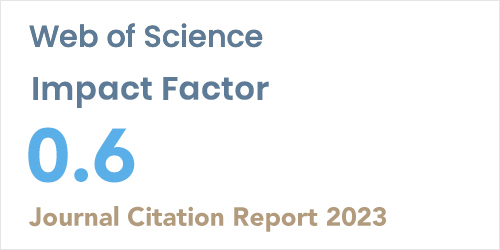ISSN: 1304-7191 | E-ISSN: 1304-7205
Volume: 41 Issue: 4 Year: 2023Comparison of Fem Solution with Analytical Solution of Continuous and Discontinuous Contact Problem
1Gumuhane University, Civil Engineering Department, GUMUSHANE
2Munzur University, Construction Technology Department, TUNCELI
3Karadeniz Technical University, Civil Engineering Department, TRABZON
Sigma J Eng Nat Sci 2018; 36(4): 977-992

#### Abstract

The continuous and discontinuous contact problem of a homogeneous layer loaded with three rigid flat blocks and resting on the elastic semi-infinite plane was investigated in this study by using the finite element method (FEM). All surfaces are assumed to be frictionless. The homogenous layer was loaded by means of three rigid flat blocks whose external loads are Q and P (per unit thickness in 𝑧 direction). Two dimensional finite element analysis of the problem was performed using ANSYS package program. Analyzes were performed for loading conditions which are Q=P, Q=2P, Q=4P, Q=8P and Q=10P, and different values of block widths, distances between blocks and shear modulus. The initial separation loads and initial separation distances ( , ) cr cr   between the homogeneous layer and the elastic semi-infinite plane were determined for continuous contact condition. The length of the separation zone was obtained for discontinuous contact condition. In addition to these, normal stress distributions between the layer and the semi-infinite plane were determined for continuous and discontinuous contact conditions. As a result of the study, the analytical results, and the results of the finite element method were shown by comparing as graphs and tables.